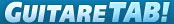Song name# A B C D E F G H I J K L M N O P Q R S T U V W X Y Z

# Lou Reed - Im So Free tab

Highlighted       Show chord diagrams
```intro   {A}{G}{D}{D}
{A}{G}{D}{D}

Verse   {D}{A/G}{C/G}{G/D}   Yes, I am mother nature\'s son, and im the
only one
{D}{A/G}{C/G}{G/D}   I do what I want and I want what I see,
you know it happens to me

chorus  {A}{G}{D}{D}         (I\'m so free
{C}{G}{D}{D}         I\'m so free

Solo    {A}{G}{D}{D}
{A}{G}{D}{D}

Verse   {D}{A/G}{C/G}{G/D}   Oh please, Saint Germaine, I have come
this way
{D}{A/G}{C/G}{G/D}   Do you remember the shape I was in, I had
horns and fins

chorus  {A}{G}{D}{D}         I\'m so free
{C}{G}{D}{D}         I\'m so free
[ Tab from: http://www.guitaretab.com/l/lou-reed/157877.html ]
Verse   {D}{D}{G}{G/D}       Do you remember the silver walks, you
used to shiver, and I used to talk
{D}{D}{G}{G/D}       Then we went down to Times Square, and
ever since then Ive been hangin round
there

chorus  {A}{G}{D}{D}         I\'m so free
{C}{G}{D}{D}         I\'m so free

Verse   {D}{A/G}{C/G}{G/D}   Yes, I am mother nature\'s son, and im the
only one
{D}{A/G}{C/G}{G/D}   I do what I want and I want what, and I
want what I see, could only happen to me

chorus  {A}{G}{D}{D}         I\'m so free
{C}{G}{D}{D}         I\'m so free, Oh-oh

Outro   {G}{D}{G}{D}         oh, I\'m so free, oh-oh-oh
{G}{D}{G}{D}         oh, I\'m so free, early in the evening
{G}{D}{G}{D}         I\'m so free, yeah
{G}{D}{G}{D}         good, I\'m so free, when it\'s ...)